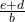## make x the subject of e = bx-d YA’LL PLEASE I CAN’T DO MATHS​

Question

make x the subject of e = bx-d

YA’LL PLEASE I CAN’T DO MATHS​

in progress 0
3 months 2021-08-22T18:57:19+00:00 2 Answers 0 views 0

1. ### Answer:  x = (e+d)/b

Explanation:

Your teacher wants you to isolate x.

We first undo the “minus d” by adding d to both sides. Then we divide both sides by b to undo the multiplication. In short, we follow PEMDAS in reverse undoing everything to isolate the variable.

So,

e = bx-d

e+d = bx

bx = e+d

x = (e+d)/b

x == x